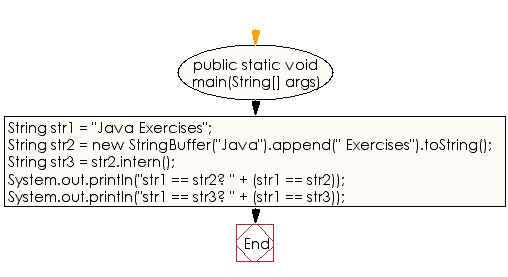﻿ Java exercises: Get the canonical representation of the string object - w3resource# Java String Exercises: Get the canonical representation of the string object

## Java String: Exercise-20 with Solution

Write a Java program to get the canonical representation of the string object.

Sample Solution:

Java Code:

``````public class Exercise20 {
public static void main(String[] args)
{
// Create three strings in three different ways.
String str1 = "Java Exercises";
String str2 = new StringBuffer("Java").append(" Exercises").toString();
String str3 = str2.intern();

// Determine which strings are equivalent using the ==
// operator (as compared to calling equals(), which is
// a more expensive operation.
System.out.println("str1 == str2? " + (str1 == str2));
System.out.println("str1 == str3? " + (str1 == str3));
}
}
```
```

Sample Output:

```str1 == str2? false
str1 == str3? true
```

Flowchart:Visualize Java code execution (Python Tutor):

Java Code Editor:

Improve this sample solution and post your code through Disqus

What is the difficulty level of this exercise?

﻿